Refer to our Texas Go Math Grade 7 Answer Key Pdf to score good marks in the exams. Test yourself by practicing the problems from Texas Go Math Grade 7 Module 4 Quiz Answer Key.

4.1 Similar Shapes and Proportions

Question 1.
Explain whether the shapes are similar.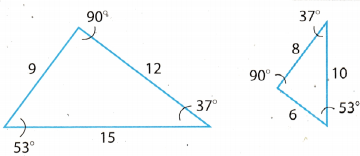Yes, both the given shape (triangle) are similar.
Because all the angLes of both triangles are equal and also the ratio of corresponding sides of the triangle are equal.
$$\frac{9}{6}$$ = $$\frac{15}{10}$$ = $$\frac{12}{8}$$ = $$\frac{3}{2}$$
Hence, both shapes are similar.

4.2 Using Similar Shapes

Find the missing measure in each pair of similar shapes.

Question 2.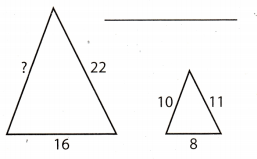These two shapes are similar, so the corresponding sides are proportional.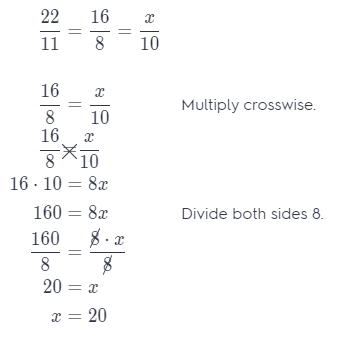The missing measure is 20.

Question 3.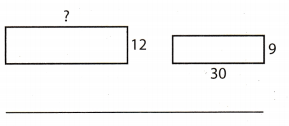\centering To find the missing measure, use a proportion.
$$\frac{30}{9}$$ = $$\frac{x}{12}$$
$$\frac{30}{9} \cdot \frac{1.33}{=1.33} \frac{x}{12}$$
$$\frac{39.9}{12}$$ = $$\frac{x}{12}$$
39.9 = x
The missing measure is x = 39.9.

4.3 Similar Shapes and Scale Drawings

Question 4.
What is the area of the room represented in the scale drawing?3cm : 6ft4 ⇔ 1 cm : 2 ft
l = 10cm
w = 6 cm
Convert length and width from cm to ft
l = 10 ∙ 2 = 20 ft
w = 6 ∙ 2 = 12 ft
Use the formula for the area of the rectangle.
A = 1 w Substitute 20 for 1 and 12 for w.
A = 20 ∙ 12
A = 240
The area of the room is 240 ft2.

4.4 Ratios and Pi

Question 5.
Find the missing measure.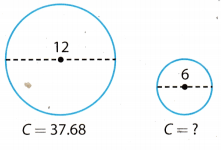C = 37.68 The circumference of big circle
d = 12 Diameter of big circle
C1 = ? The circumference of similar circle
d1 = 6 Diameter of similar circle
\centering To find the missing measure, use a proportion.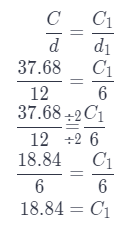The circumference of similar circle is 18.84.

Essential Question

Question 6.
How can you use similarity and proportionality to find missing measures?
For every similar shapes, the corresponding measures are proportional so from proportion we can find the missing measures.

Texas Go Math Grade 7 Module 4 Mixed Review Texas Test Prep Answer Key

Selected Response

Question 1.
Which shows a pair of shapes that are not similar? All corresponding angles have equal measure.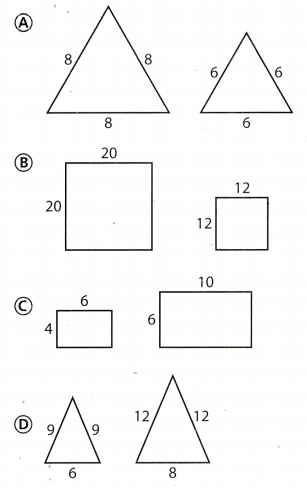Example under (C) shows a pair of shapes that are not similar.

Explanation:
For value of side from the picture, when we write a proportion using corresponding sides, we get;
(A) $$\frac{8}{6}=\frac{8}{6}=\frac{8}{6}$$
which is correct

(B) $$\frac{20}{12}=\frac{20}{12}$$
which is correct

(C) $$\frac{4}{6}=\frac{6}{10}$$
0.66 = 0.6
which isn’t correct

(D) $$\frac{9}{12}=\frac{9}{12}=\frac{6}{8}$$
0.75 = 0.75 = 0.75
which is correct

Question 2.
A scale drawing of a rectangular deck is shown below.What is the perimeter of the actual deck?
(A) 187.5 ft
(B) 375 ft
(C) 550 ft
(D) 750 ft
(B) 375 ft

Explanation:
2 in : 25 ft ⇔ 1 in : 12.5 ft
Convert Length and width from in. to ft.
l = 11 ∙ 12.5 = 137.5 ft
w = 4 ∙ 12.5 = 50 ft
Use the formula for the perimeter of rectangle.
P = 2(l + w) Substitute 137.5 for l and 50 for w.
P = 2(137.5 + 50)
P = 375
The perimeter of the actuaL deck is 375 ft

Question 3.
The two triangles below are similar. Find the area of the larger triangle.(A) 8 square units
(B) 36 square units
(C) 64 square units
(D) 128 square units
The area of the larger triangle is (C) 64 square units.

Explanation:
x1 = 6
y1 = 12
x = ?
y = 16
\centering To find the missing measure, use a proportion.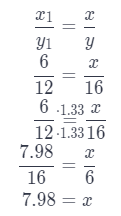The area of the larger triangle is:
$$\frac{7,98 \cdot 16}{8}$$ = 63.84 ≈ 64

Question 4.
Find the circumference of the smaller circle.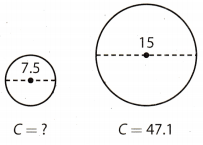(A) 15.7
(B) 22.5
(C) 23.55
(D) 31.4
(C) 23.55

Explanation:
C1 = the circumference of the larger circle
C2 = the circumference of the smaller circle
d1 = the diameter of the larger circle
d2 = the diameter of the smaller circle
Two circles are similar, so the corresponding measures are proportional.
Write the proportion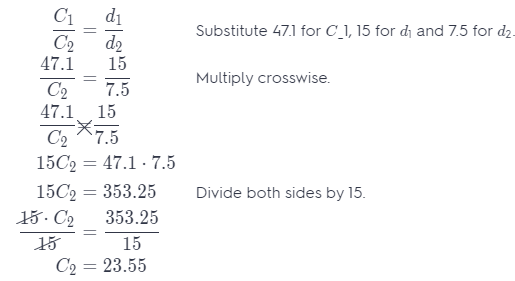The circumference of the smaller circle is 23.55.

Gridded Response

Question 5.
An advertising company is creating a large wall banner and a smaller flyer that are similar figures. What percent of the area of the banner is the area of the flyer?x1 = 15
y1 = 40
x = ?
y = 24
\centering To find the missing measure, use a proportion.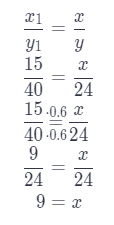Use formula for the area of rectangLe P = x ∙ y where x represents Length of rectangle and y represents width of rectangle.
The area of a large wall banner is:
P1 = 15 ∙ 40 = 600
The area of smaller flyer is:
P = 9 ∙ 24 = 216
When we divide the area of smaller flyer by the area of a large wall banner, we get which percent of the area of the banner is the area of a smaller flyer.
$$\frac{216}{600}$$ = 0.36 = 36%

Scroll to Top